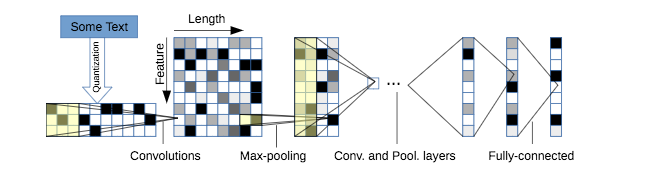# How to partially load a model for transfer learning

Hi everyone,

I have a (probably basic) question.

I have found a pytorch model on github which I want to use. Link. The model implemented on this repository is represented in the following figure:I first train model with the dataset given in the repository and then I want to finetune it in my dataset which have different number of classes. Therefore, I want to load weights of all layers except the last fully connected layer. Is there a way to do that ? If I want to load the complete model it is done as follows:

``````

``````

Model architecture as follows:

``````
def forward(self, x):
x = self.conv1(x)
x = self.conv2(x)
x = self.conv3(x)
x = self.conv4(x)
x = self.conv5(x)
x = self.conv6(x)

# collapse
x = x.view(x.size(0), -1)
# linear layer
x = self.fc1(x)
# linear layer
x = self.fc2(x)
# linear layer
x = self.fc3(x)
# output layer
x = self.log_softmax(x)

return x
``````

I just want to ignore the self.fc3. In the original trained model it is `self.fc3 = nn.Linear(1024, 4)` but in my model it will be `self.fc3 = nn.Linear(1024, 6)` therefore I want that part to be randomly initialized.

Is it possilbe to do that ? If so, could you help me ?

EDIT

Actually I know that, I can do the following in order to reach the individual layers’ weights:

``````>>>>checkpoint = torch.load(args.model_path)
>>>>checkpoint['state_dict'].keys()
odict_keys(['conv1.0.weight', 'conv1.0.bias', 'conv2.0.weight', 'conv2.0.bias', 'conv3.0.weight', 'conv3.0.bias', 'conv4.0.weight', 'conv4.0.bias', 'conv5.0.weight', 'conv5.0.bias', 'conv6.0.weight', 'conv6.0.bias', 'fc1.0.weight', 'fc1.0.bias', 'fc2.0.weight', 'fc2.0.bias', 'fc3.weight', 'fc3.bias'])
>>>> newmodel = charCNN(args) # How to use odict_keys to initialize weights of newmodel with checkpoint?
``````

However, I do not know how can I exclude the fc3.weight and fc3.bias and assign the other weights to the corresponding fields in the new model automatically.